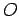# What is Hermitian, Operator or Eigenfunction?##What is Hermitian, Operator or Eigenfunction?

What is considered Hermitian, the operators (x, -i*(h/2π)*d/dx, H^, etc.) or the Wave Function (ψ) itself? Many texts suggest that there are Hermitian Operators, but no one says that the wave function is hermitian.
By the way, a Hermitian operator, as given in various sources, satisfies the property :
(Integral,-inf, inf)fA^g = (Integral,-inf,inf)gA^f, i.e., A^ = A^*.

##Re: What is Hermitian, Operator or Eigenfunction?

Operators are Hermitian not the wave-function.
A notation:

<ψ₁|O|ψ₂>        ≡ ∫ {ψ₁*(O ψ₂)} dx from -∞ to ∞.

There is a quantity called Hermitian Conjugate of any operator.Let us for the moment denote it as.It satisfies
<ψ₁ | O ψ₂> = <ψ₁ | ψ₂>

Now if=Thenis called a Hermitian Operator.Permissions in this forum:
You cannot reply to topics in this forum